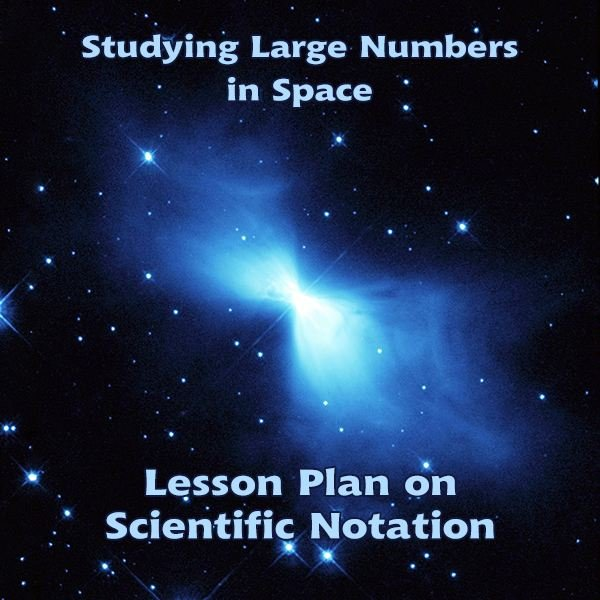A Lesson on Scientific Notation for Grade 8: Studying Large Numbers in SpaceYou are an astronomer studying asteroids in space. In your study of asteroids, you will use scientific notation to estimate and compare distances and sizes of asteroids.

Lesson Objective: Lesson is aligned to the Common Core State Standards for Mathematics – 8.EE.3 Expressions and Equations – Use numbers expressed in the form of a single digit times an integer power of 10 to estimate very large or very small quantities, and to express how many times as much one is than the other. Lesson is aligned to the Common Core State Standards for Mathematics – 8.EE.4 Expressions and Equations – Perform operations with numbers expressed in scientific notation, including problems where both decimal and scientific notation are used. Use scientific notation and choose units of appropriate size for measurements of very large or very small quantities. Interpret scientific notation that has been generated by technology.

Lesson ProcedureOne of the largest asteroids, Amphitrite, was discovered in 1854. The estimated distance of Amphitrite to the sun is recorded to be 382,100,000 km. What is the estimate of the distance of Amphitrite to the sun written in scientific notation?

1. To write this number in scientific notation, move the decimal point so that the number lies between 1 and 10.
2. To write 382,100,000 in scientific notation, move the decimal point 8 places to the left.
3. To write very large numbers in scientific notation, positive integer exponents are used when the decimal point is moved to the left.
4. The large number 382,100,000 is written in scientific notation as 3.821 x 108. The exponent is 8 because the decimal point was moved 8 places to the left.

The asteroid, Camilla, was discovered in 1868. The estimated distance of Camilla to the sun is recorded to be 521,800,000 km.

5. What is the estimate of the distance of Camilla to the sun written in scientific notation? Explain the steps you used to write the number in scientific notation.

Answer: 5.218 x 108. I moved the decimal point 8 places to the left so that the number is between 1 and 10. I used 8 as the exponent because I moved the decimal point to the left 8 places.

Large distances in space are measured in “astronomical units”, AU. One AU is the estimated distance from the Sun’s center to the Earth’s center. An AU is equal to 149,597,871 km or approximately 1.5 x 108.

6. The distance of the asteroid Chiron to the Sun is 13.72 AU. What is the approximate distance in kilometers of Chiron to the Sun? Write your answer in scientific notation. Show or explain how you found your answer.

Answer: I multiplied 1.5 x 108 x 13.72 = 2.058 x 109